Saturday , November 28 2020# 10th Class CBSE Mathematics 2018-19

## 10th Class CBSE Mathematics 2018-19

Time: 3 hours
M.M.: 80
Class: 10th
Subject: Mathematics
Date: 07/03/2019

## General Instructions:

• All questions are compulsory.
• This question paper consists of 30 questions divided into four sections – A, B, C and D.
• Section A contains 6 questions of 1 mark each. Section B contains 6 questions of 2 marks each. Section C contains 10 questions of 3 marks each. Section D contains 8 questions of 4 marks each.
• There is no overall choice. However, an internal choice has been provided in two questions of 1 marks two questions of 2 marks, four questions of 3 marks each and three questions of 4 marks each. You have to attempt only one of the alternative in all such questions.
• Use of calculator is not permitted.

## Section A

### Question numbers 1 to 6 carry 1 mark each.

#### Question: 3. In fig. 1, DE||BC, AD = 1 cm and BD = 2 cm. What is the ratio of the ar (Δ ABC) to the ar (Δ ADE)?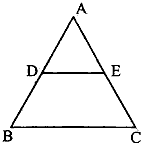## Section C

### Question number 13 to 22 carry 3 marks each.

#### Question: 14. PQ is a chord of length 8 cm of a circle of radius 5 cm. The tangents at p and Q intersect at a point T (see Fig. 2). Find the length TP.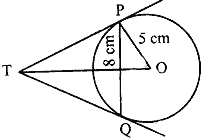#### Question: 19. Find the made of the following frequency distribution.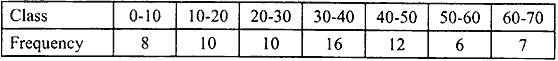#### Question: 21. In fig. 3, ∠ ACB = 90° and CD⊥ AB, prove that CD² = BD × AD.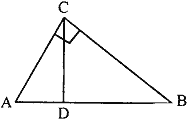#### Question: 22. Find the area of the shaded region in Fig. 4, if ABCD is a rectangle with sides 8 cm and 6 cm and O is the centre of circle. (Take π = 3.14)#### Question: 25. The following distribution gives the daily income of 50 workers of a factory.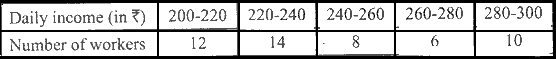#### Convert the distribution above to a ‘less than type’ cumulative frequency distribution and draw its ogive. Or The table shows the daily expenditure on food of 25 households in a locality. Find the mean daily expenditure on food.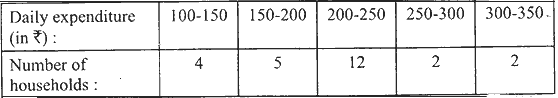## 9th CBSE Social Studies Examination: Mid Term 2019

9th CBSE Social Studies Examination: Mid Examination Question Paper (2019-20) School Name: Himalaya Public School, Sector …